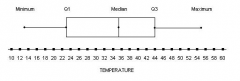• Shuffle
Toggle On
Toggle Off
• Alphabetize
Toggle On
Toggle Off
• Front First
Toggle On
Toggle Off
• Both Sides
Toggle On
Toggle Off
Toggle On
Toggle Off
Front

### How to study your flashcards.

Right/Left arrow keys: Navigate between flashcards.right arrow keyleft arrow key

Up/Down arrow keys: Flip the card between the front and back.down keyup key

H key: Show hint (3rd side).h key

A key: Read text to speech.a keyPlay buttonPlay buttonProgress

1/41

Click to flip

### 41 Cards in this Set

• Front
• Back
 the researcher merely observes what ishappening or what has happened in the past and tries to draw conclusions basedon these observations Observational Study the researcher manipulated one of thevariables and tries to determine how the manipulation influences othervariables Experimental Study a characteristic or measure obtained by using the data values from a sample. Statistic a characteristic or measure obtained by using all the data values from a specific population Parameter consists of all subjects being studied Population a group of subjects from the population Sample the organization of raw data in table form, using classes and frequencies Frequency Distribution raw data values are placed into a quantitative or qualitative category called a class Class limits the largest and smallest value that can be included in that class Upper and lower class limits the number of values in each class Frequency numbers used to separate the classes so that there are no gaps in the frequency distribution Class boundaries is found by subtracting the lower (or upper) class limit of one class from the lower (or upper) class limit of the next class Class width Class midpoint Xm= (Lower Limit+Upper limit)/2 shows the number of data values that are less than or equal to a specific value ; found by adding the frequencies of the classes less than or equal to the upper class boundary of a specific class ; shows the number of data values that are less than or equal to a specific value ; found by adding the frequencies of the classes less than or equal to the upper class boundary of a specific class D Cumulative frequency distribution when the range of data values is very small a frequency distribution can be constructed using single data values for each class Ungrouped Frequency distribution a graph that displays the data by using contiguous vertical bars (unless the frequency of the class is 0) of various heights to represent the frequency of the classes Histogram a graph that displays the data by using lines that connect points plotted for the frequencies at the midpoints of the classes. The frequencies are represented in the heights of the points frequency polygon (aka the cumulative frequency graph) is a graph that represents the cumulative frequencies of the classes in a frequency distribution Ogive Variance Equation σ^2=(Σ(X−μ))/N Standard deviation equation σ=√((Σ(X−μ))/N) Q2= Median Q1= median for all values that fall below the median Q3= median for all the data values that are above the median IQR= Q3-Q1Box plot a graph of a data set obtained by drawing a horizontal line from the minimum data value to Q1 , drawing a horizontal line from Q3 to the maximum data value, and drawing a box whose vertical sides pass through Q1 and Q3 with a vertical line inside the box passing through the median box plot cannot occur at the same time (have no outcomes in common) Mutually Exclusive events The chance of an event occurring Probability the probability of event E is a number (either a fraction or a decimal) between and including 0 and 1. This is denoted by 0≤P(E) ≤ 1 Probability rule 1 If an event E can occur (i.e., the event contains no members in the same sample space), its probability is 0. Probability Rule 2 If an event E is certain, then the probability of E is 1. Probability Rule 3 The Sum of the Probabilities of all the outcomes in the sample space is 1 Probability Rule 4 Ρ(Ē)=1−P(E) P(E)=1−P(Ē)P(E)+P(Ē)=1 Probability rule for complementary events Formula for emprical probability P(E)= (frequency for the class)/(total frequencies in the distribution)=f/n� distribution)=f/n Properties of a Binomial experiment Fixed number of trials Each trial can only have two outcomes or outcomes that can be reduced to two outcomes. These can be considered either a success or a fail The outcomes of each trial must be independent of one another The probability of a success must remain the same for each trial the outcomes of a binomial experiment Binomial distribution a continuous, symmetric, bell-shaped distribution of a variable Normal Distribution Properties of Normal Distribution Bell shaped Mean, median, and mode are located at the center Unimodal The curve is symmetric about the mean computed from the same sample data measures the strength and direction of a linear relationship between two quantitative variables. The symbol for the population correlation coefficient is ρ (Greek letter rh�) Correlation Coefficient Equation for Correlation Coefficient r=(n(Σxy)−(Σx)(Σy))/√([n(Σx^2 )−(Σx)^2 ][n(Σy^2 )−(Σy)^2]) a statistical test for the mean of a population. It can be used when n≥30 or when the population is normally distributed and σ is known Z-test